﻿ SPF规格材弹性模量的动态测试及其概率分布
 林业科学2015, Vol. 51Issue (2): 105-111PDF
DOI: 10.11707/j.1001-7488.20150213
0

#### 文章信息

Wang Zheng, Gu Lingling, Gao Zizhen, Liu Bin
SPF规格材弹性模量的动态测试及其概率分布
Dynamic Testing and Probability Distribution of Elastic Modulus of SPF Dimension Lumbers

Scientia Silvae Sinicae, 2015, 51(2): 105-111.
DOI: 10.11707/j.1001-7488.20150213

### 作者相关文章

SPF规格材弹性模量的动态测试及其概率分布

1. 南京林业大学材料科学与工程学院 南京 210037；
2. 南京林业大学机械电子工程学院 南京 210037

Dynamic Testing and Probability Distribution of Elastic Modulus of SPF Dimension Lumbers
Wang Zheng1, Gu Lingling2, Gao Zizhen1, Liu Bin2
1. College of Materials Science and Engineering, Nanjing Forestry University Nanjing 210037;
2. College of Mechanical and Electronic Engineering, Nanjing Forestry University Nanjing 210037
Abstract: [Objective] SPF dimension lumber is a principal material for light-frame timber structures. In this paper, modulus of elasticity (MOE) of SPF dimension lumbers was accurately measured by frequency method. The feasibility of the measured data was studied by Weibull distribution. Then, the data was fitted linearly and verified by K-S method. Meanwhile, a series of possible Weibull parameters was obtained by linear fitting of MOE data in Weibull coordinate. [Method] We randomly extract SPF dimension lumbers with capacity of 300 from Nanjing Cogent Home Manufactory Ltd. We realize free beam of test samples by supporting structure hung vertically and freely with elastic rope. The accuracy of free beam realization is proved by the result of modal test. Under transient excitation, the elastic modulus value of the samples is obtained by testing their first transverse bending frequency. The correctness of the test results of frequency method is proved by stress wave method. To exactly evaluate the quality of SPF dimensional lumbers, the testing data was deeply studied by using methods such as Weibull distribution, K-S method in normal distribution, calculation of probability data of MOE value under given values and probability distribution method. [Result] A good correlation was found between elastic modulus tested with frequency method and that tested with wave-speed method. As can be seen from the K-S testing results of Weibull distribution and normal distribution, the elastic modulus of SPF dimension lumbers is not subjected to two-parameter Weibull distribution (Eu=0), but it is subjected to three-parameter Weibull distribution, in which location parameter Eu is larger than half of the minimum test value. By analyzing the probability data of MOE value under given values for the total SPF dimension lumbers, the calculated probability values are stable and similar when using 3 500, 4 000 and 4 500 MPa as the given value of Eu. In this case, the given values of Eu are 63%, 72% and 81% of the minimum value of elastic modulus (5 526 MPa), respectively. Therefore, it is recommended to use the value that is 60%-80% of the minimum actual data as the given value of location parameter Eu in three-parameter Weibull distribution. Probabilities for elastic modulus of SPF dimension lumbers calculated with given value in three-parameter Weibull distribution and normal distribution are equal. According to the probability distribution curve and density curve, probability density in Weibull is slightly asymmetric to its mean value (9 997 MPa), which is different from probability density curve in normal distribution; Probability curve in normal distribution is a little higher than that in Weibull distribution when elastic modulus is less than 7 000 MPa. However, the results would opposite when the elastic modulus is more than 7 000 MPa. [Conclusion] Modal test verifies testing unit in the work and realizes the supporting conditions for free hanging of test lumbers. Test schemes of frequency method are put forward to ensure the testing precision of natural frequency of the first bending mode and reliably calculate elastic modulus of SPF dimension lumbers; Testing elastic modulus of SPF dimension lumbers by instantaneous aroused frequency method has virtues of convenience, fast, repeatability and accurate; A good correlation was found between elastic modulus tested with frequency method and that tested with wave-speed method. Such a correlation verifies correctness of elastic modulus tested with frequency method. Results tested by the K-S test method showed that general elastic modulus of SPF dimension lumbers obeyed three-parameter Weibull distribution and normal distribution, but not obeyed two-parameter Weibull distribution. Probability of elastic modulus calculated in normal distribution, which is less than 7 000 MPa, is higher than that calculated in Weibull distribution. In other scope, however, probabilities calculated in these two distribution ways are identical; Probability density curve for overall elastic modulus of SPF dimension lumbers in three-parameter Weibull distribution is slightly asymmetric; It is recommended to use 60%-80% of the minimum test data as location parameter Eu when the parameters of Weibull distribution is determined. The overall average and standard deviation of MOE values of total SPF dimension lumbers were calculated. The probabilities of MOE values under given values were also calculated. As a result, the calculated probabilities were same, except the fact that probabilities calculated by normal distribution were higher than those calculated by Weibull distribution when the MOE values were within the scope of low end. To evaluate the quality of these SPF dimension lumbers, the methods of three-parameters Weibull distribution and normal distribution were particularly used to calculate the probability that general MOE value was less than 8 000 MPa (the minimum standard value for Canadian No.2 lumbers), and the probability values were 13.8% and 13.6% respectively.
Key words: spruce, pine and fir    modulus of elasticity    frequency    stress wave    Weibull distribution    normal distribution

SPF规格材是轻型木结构建筑中的主材，其弹性模量是衡量材料产生弹性变形难易程度的指标(郑栋梁等，2002)，因此SPF规格材弹性模量测定及分析尤为重要(Linda et al., 1997Zhu et al., 2010)。为提高对弹性模量的检测水平以及对弹性模量数据的统计分析范围，笔者从南京北美木屋有限公司一批SPF规格材中随机抽取容量为300的样本作为试件，采用弹性绳垂直自由悬挂方式的支承结构对试件实现自由梁，模态试验验证了自由梁的精度。在瞬态激励下，通过测试试件一阶弯曲频率，得到其弹性模量(王正，2007)； 应用应力波法验证了频率法测试结果的正确性(Raini et al., 2000)。 测试数据的精确性是数据处理正确性的前提。一般地，数据统计分析应用最多的是正态分布，本文除应用频率法精确地测量弹性模量外，还探讨应用威布尔分布来分析SPF规格材弹性模量数据的可行性。根据实测的子样弹性模量数据，对其进行直线拟合和K-S法检验，结果表明SPF规格材弹性模量除服从三参数威布尔分布外，还服从正态分布。最后，根据加拿大SPF规格材二级材弹性模量在8 000～9 000 MPa标准，借助威布尔分布和正态分布分别推算了总体二级规格材达标的概率。

1 瞬态激励频率法测量SPF规格材弹性模量 1.1 测试原理

 $E = 0.946\frac{{\rho f_1^2{L^4}}}{{{h^2}}}$ (1)

1.2 试件和测试方法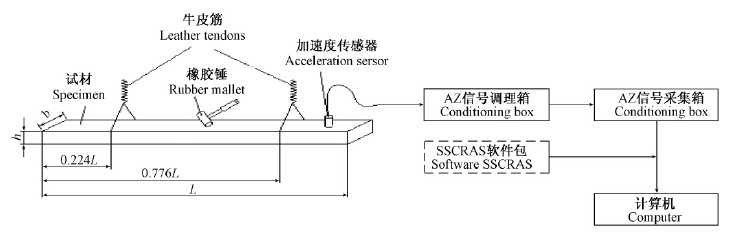图 1 SPF试件的基频测试系统 Fig. 1 Testing system flow chart of natural frequency of SPF testing lumbers
1.3 试验方案

1)采集参数设置: 分析频率设为50 Hz，FFT长度为2 048(频率测试精度可达0.062 5 Hz)，采集方式为自由运行，数据块1块，电压范围 ±1 250 mV(程控放大4倍)，调理仪放大倍率设为100。滤波频率设为50 Hz，防止频率混迭，以保证在频谱上不会出现三阶固有频率的混迭频率(假频率)。

2)加速度计安装于试件左端或右端，橡胶锤敲击试件跨中。跨中是二阶弯曲振型节点位置，使得在50 Hz分析频率的频谱图上不出现二阶频率的峰值。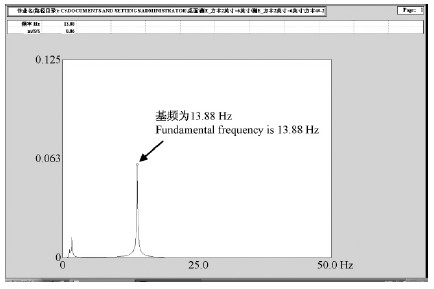图 2 SPF规格材的26号试件测量频谱 Fig. 2 Measured spectrum of No.26 piece of SPF dimension lumbers
2 测试结果及其验证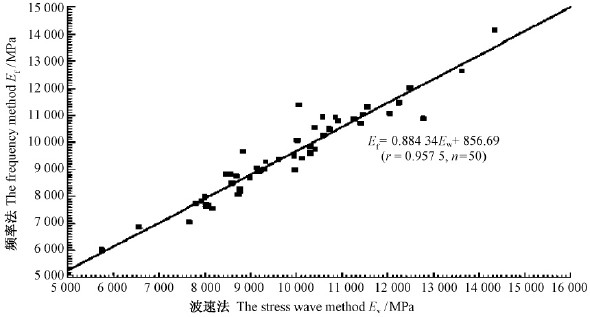图 3 频率法-应力波法测量弹性模量的相关关系 Fig. 3 Correlativity of measuring MOE value by the frequency method and stress wave method

3 SPF规格材弹性模量概率分布 3.1 Weibull分布及其数字特征(Weibull et al., 1951)

 $\begin{gathered} \Pr {\text{o}}\left({\zeta \leqslant E} \right)= F\left(E \right)= 1 - \exp \left[ { - {{\left({\frac{{E - {E_{\text{u}}}}}{{{E_0}}}} \right)}^m}} \right]，\hfill \\ E{\text{ > }}{E_{\text{u}}}; \hfill \\ \Pr {\text{o}}\left({\zeta \leqslant E} \right)= F\left(E \right)= 0，E{\text{ < }}{E_{\text{u}}} \hfill \\ \end{gathered}$ (2)

 $\mu = {E_0}\Gamma \left({1 + \frac{1}{m}} \right)+ {E_{\text{u}}}$ (3)
 $\sigma = {E_0}\sqrt {\Gamma \left({1 + \frac{2}{m}} \right)- {\Gamma ^2}\left({1 + \frac{1}{m}} \right)}$ (4)

3.2 Weibull分布拟合检验

 $P\left({\zeta \leqslant {E_i}} \right)= 1 - \exp \left[ { - {{\left({\frac{{{E_{\text{i}}} - {E_{\text{u}}}}}{{{E_0}}}} \right)}^m}} \right]$

 $\lg \left({\lg \frac{{n + 1}}{{n + 1 - i}}} \right)= m\lg \left({{E_i} - {E_u}} \right)+ \lg \left({\frac{{\lg e}}{{E_0^m}}} \right)$ (5)

Eu=4 500 MPa时，试验点对在威布尔坐标系中的散点图如图 4所示。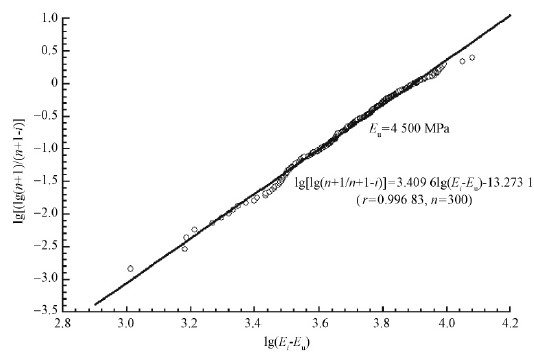图 4 SPF规格材弹性模量威布尔分布的拟合检验 Fig. 4 Fitting inspection schedule of Weibull distribution with MOE values of SPF dimension lumbers

Eu=4 500 MPa时，测量点用直线拟合的相关系数r=0.996 83，n=300，拟合效果好，故SPF规格材弹性模量在威布尔坐标系中可以用一条直线拟合。

 $F\left(E \right)= P\left({\zeta \leqslant E} \right)= 1 - \exp \left[ { - {{\left({\frac{{E - 4500}}{{6118}}} \right)}^{3.4096}}} \right]$ (6)

 $\begin{gathered} f\left(E \right)= 5.5731 \times {10^{ - 4}}{\left({\frac{{E - 4500}}{{6118}}} \right)^{2.4096}} \hfill \\ \exp \left[ { - {{\left({\frac{{E - 4500}}{{6118}}} \right)}^{3.4096}}} \right] \hfill \\ \end{gathered}$ (7)

m=3.409 6，E0=6 118 MPa，Eu=4 500 MPa代入式(3)和式(4)，得总体平均值μ和标准离差σ的估计值为 μ=9 997 MPa，σ=1 781 MPa；变异系数为17.8%。容量为300样本，样本平均值为10 001 MPa，标准离差为1 822 MPa。3.3 威布尔分布和正态分布的K-S检验Dn的临界值Dn，α由样本容量和显著性水平α查表获得(吴世伟等，1990)。

α=0.05，n=300，在参数未知时，Dn，α=D300，0.05=0.888/$\sqrt {300}$=0.051 27。

α=0.05，n=300，在参数未知时，Dn，α=D300，0.05=0.886/$\sqrt {300}$=0.051 15。

3.4 SPF规格材总体弹性模量在给定值下的概率计算1)以3 500，4 000，4 500 MPa为Eu的设定值时，计算的概率值稳定且基本相同，这时Eu设定值分别是弹性模量测试的最小值5 526 MPa的63%，72%和81%。因此，运用威布尔分布进行SPF规格材弹量模量概率计算时，推荐采用根据实测数据最小值的60%～80%作为位置参数Eu设定值的三参数威布尔分布。

2)三参数威布尔分布和正态分布计算的SPF规格材弹性模量在给定值下的概率相等(精确到小数点后的第3位)。

SPF规格材弹性模量的概率分布曲线和概率密度曲线，可清晰地说明三参数威布尔分布和正态分布计算的概率特点(图 5)。从图 5可以看到：1)威布尔概率密度相对于它的均值(9 997 MPa)略显不对称性，不同于正态概率密度曲线相对于均值是对称的；2)弹性模量不超过7 000 MPa时，正态概率分布曲线略高于威布尔概率分布曲线，超过7 000 MPa后，则正好相反。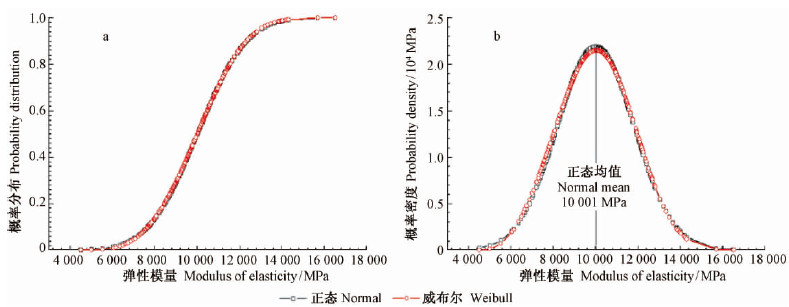图 5 SPF规格材弹性模量的概率分布曲线(a)和概率密度曲线(b) Fig. 5 Probability distribution curve(a) and probability density curve(b) of MOE value of SPF dimension lumbers
3.5 SPF规格材质量评价

4 结论

1)模态试验验证了文中的试验装置实现了试件自由悬挂的支承条件，提出了频率法的测试方案，保证了一阶弯曲振动固有频率测试精度，可靠地推算出SPF规格材的弹性模量。

2)瞬态激励频率法测试SPF规格材的弹性模量具有简便、快速、重复性好和精度高等优点。

3)频率法和波速法测出的弹性模量具有较好的相关性，验证了频率法测试弹性模量的正确性。

4)SPF规格材总体弹性模量不服从两参数威布尔分布。

5)SPF规格材总体弹性模量服从三参数威布尔分布，在确定威布尔分布参数时，推荐按最小试验数据的60%~80%设定位置参数Eu值。

6)SPF规格材总体弹性模量的三参数威布尔概率密度曲线相对于均值略显非对称性。

7)SPF规格材总体弹性模量除服从三参数威布尔分布外，还服从正态分布。正态分布计算的弹性模量不超过

7 000 MPa的概率高于威布尔分布的计算值，在其他范围，2种分布计算的概率值是相同的。

8)应用三参数威布尔分布和正态分布推断SPF规格材总体的弹性模量达不到加拿大二级规格材所规定的下限标准值，即8 000 MPa的概率分别为13.8%和13.6%。

  傅志方. 2002.振动模态分析与参数辨识. 北京: 机械工业出版社, 2-56.(Fu Z F. 2002. Modal analysis of vibration and parameter identification. Beijing:China Machine Press, 2-56.[in Chinese])(1)  李云雁, 胡传荣. 2004.设计与数据处理. 北京: 化学工业出版社.(Li Y Y, Hu C R. 2004. Design and data processing. Beijing: Chemical Industry Press.[in Chinese])(1)  提摩盛科. 1965.机械振动学.北京: 机械工业出版社, 316-333.(Timoshenko S. 1965. Mechanical vibrational science. Beijing:China Machine Press, 316-333.[in Chinese])(1)  王正. 2007.两种木质复合材料弹性模量与阻尼比的动态测量.南京林业大学学报, 31(3): 147-149. (Wang Z. 2007. Dynamic measure of elasticity model and damp ratio to HDF and OSB. Journal of Nanjing Forestry University, 31(3):147-149.[in Chinese])(1)  吴世伟, 叶 军. 1990.参数未知的K-S法检验临界值分析.港工技术, (1): 16-20(Wu S W, Ye J. 1990. The unknown parameters of the K-S method to test the critical value analysis. Port Engineering Technology, (1): 16-20.[in Chinese])(1)  张令弥. 1992.振动测试与动力分析. 北京: 航空工业出版社, 123-230.(Zhang L M. 1992. Vibration measurement and dynamic analysis. Beijing:Aircraft Industry Press, 123-230.[in Chinese])(1)  郑栋梁, 李中付, 华宏星. 2002. 结构早期损伤识别技术的现状和发展趋势. 振动与冲击, (2): 1-6.(Zheng D L, Li Z F, Hua H X. 2002. Current situation and development trend of early structural damage identification technology.Vibration and Shock, (2):1-6[in Chinese]).(1)  Gumbel E J. 1958.Statistics of Extremes. New York: Calumbia University Press.(1)  Ilic J. 2003.Dynamic MOE of 55 species using small wood beams. Holz Roh Werkst, 61(3): 167-172.(1)  Linda S B, Donald A D, et al. 1997. Mechanically graded lumber: the grading agency perspective. Wood Design Focus, Summer, 3- 6(1)  Raini C R, Francis G W, Tnomas M G, et al. 2000.Sress-wave analysis of douglas-fir logs for veneer properties. Forest Products Journal, 50(4): 49-52(1)  Wang X P, Carter P, Ross R J, et al. 2007.Acoustic assessment of wood quality of raw forest materials—a path to increase profitability. Forest Products Journal, 57(5): 6-14. (1)  Weibull W, Swenden S. 1951.A statistical distribution of function wide applicability. J of Applied Mechanics, 18: 293-297.(1)  Wang Z, Li L, Gong M. 2012.Dynamic modulus of elasticity and damping ratio of wood-based composites using a cantileverbeam vibration technique. Construction & Building Materials, 28(1): 831-834.(2)  Zhu X D, Wang F H, Cao J, et al. 2010.Nondestructive test system for wood vibration based on virtual instrument. Journal of Northwest Forestry University, 25(5): 182-186.(1)